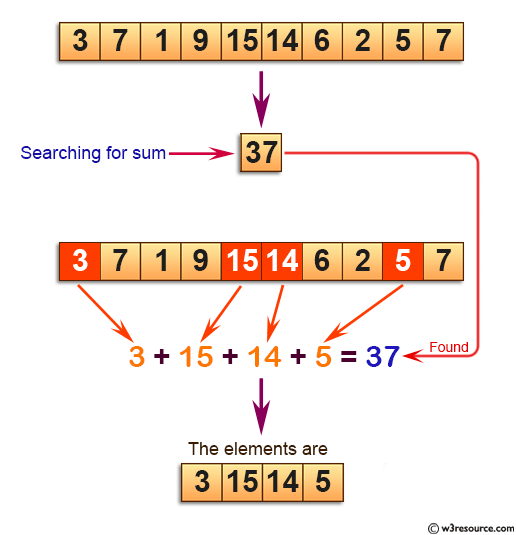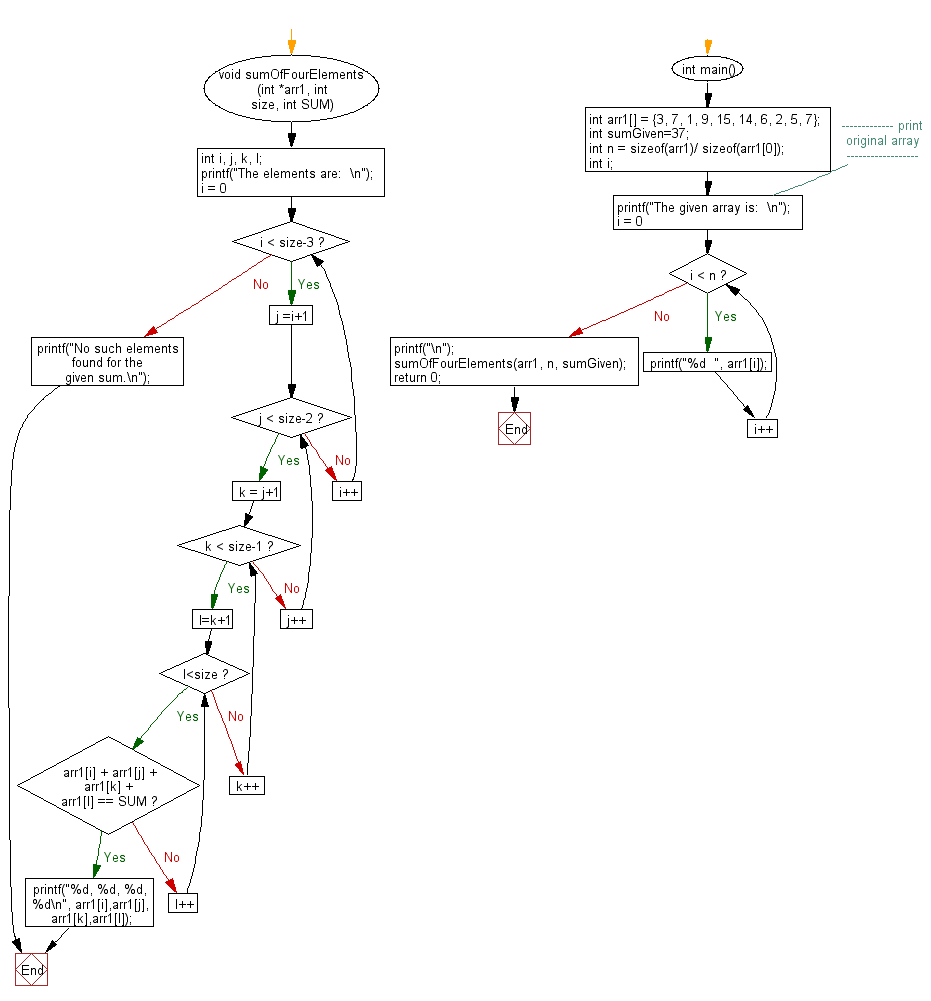﻿ C exercises: Find four array elements whose sum is equal to given number - w3resource# C Exercises: Find four array elements whose sum is equal to given number

## C Array: Exercise-78 with Solution

Write a program in C to find four array elements whose sum is equal to given number.

Pictorial Presentation:Sample Solution:

C Code:

``````#include <stdio.h>
void sumOfFourElements (int *arr1, int size, int SUM)
{
int i, j, k, l;
printf("The elements are:  \n");
for(i = 0; i < size-3; i++)
{
for(j =i+1; j < size-2; j++)
{
for(k = j+1; k < size-1; k++)
{
for(l=k+1; l<size; l++)
{
if(arr1[i] + arr1[j] + arr1[k] + arr1[l] == SUM)
{
printf("%d, %d, %d, %d\n", arr1[i],arr1[j],arr1[k],arr1[l]);
return;
}
}
}
}
}
printf("No such elements found for the given sum.\n");
}

int main() {
int arr1[] = {3, 7, 1, 9, 15, 14, 6, 2, 5, 7};
int sumGiven=37;
int n = sizeof(arr1)/ sizeof(arr1);
int i;
//------------- print original array ------------------
printf("The given array is:  \n");
for(i = 0; i < n; i++)
{
printf("%d  ", arr1[i]);
}
printf("\n");
//------------------------------------------------------
sumOfFourElements(arr1, n, sumGiven);
return 0;
}
```
```

Sample Output:

```The given array is:
3  7  1  9  15  14  6  2  5  7
The elements are:
3, 15, 14, 5
```

Flowchart:C Programming Code Editor:

Improve this sample solution and post your code through Disqus.

What is the difficulty level of this exercise?

﻿